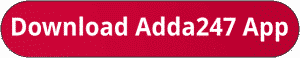Latest Banking jobs   »   IBPS quant

# Quantitative Aptitude Quiz For IBPS Clerk Prelims 2023 -30th July

Direction (1–5): Solve the given two equations and mark the correct option based on your answer.
(a) if x>y
(b) if x≥y
(c) if x<y
(d) if x ≤y
(e) if x = y or no relation can be established between x and y.Q6.A train can cross a pole in 15 seconds and travelling at the same speed it can cross a bridge of 500 m in 45 seconds, then find the length of the train?(in metre)
(a) 250
(b) 300
(c) 200
(d) 240
(e)320

Q7. A boat goes 220 km downstream and 108 km upstream in 20 hr. Speed of the boat in still water is 4 times the speed of the stream. Find the sum of time taken by the boat to go 40 km in downstream and 48 km upstream?
(a) 8 hrs
(b) 10 hrs
(c) 6 hrs
(d) 9 hrs
(e) None of these

Q8. The perimeter of a square is double than the perimeter of a rectangle. The area of the rectangle is 36 sq.cm. what is the area of square?
(a) 72 sq.cm
(b) 56 sq.cm
(c) 64 sq.cm
(d) can’t be determined
(e) 108 sq.cm

Q9. If ratio of time periods of investment of P and Q is 4:5, profit at the end of the year is 75000 and P’s share is Rs 15000, then what is the ratio of Q’s and P’s investment?
(a)5:16
(b) 6:7
(c) 12:13
(d) 16:5
(e)8:5

Q10. In how many different ways can the letter of word ‘champion’ be arranged so that all the vowels come together?
(a)4820
(b) 4320
(c) 4640
(d) 5280
(e)None of these

Solutions.                                      .                .

## FAQs

### What is the selection process of IBPS Clerk 2023

The selection process of IBPS Clerk includes Prelims and Mains exam

#### Congratulations!Union Budget 2023-24: Free PDF